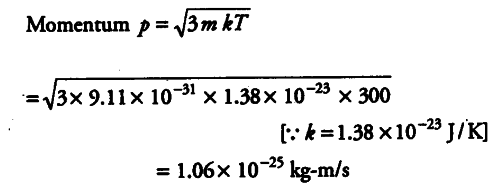# Compute the typical de-Broglie wavelength

Compute the typical de-Broglie wavelength of an electron in a metal at 27 °C and compare it with the mean separation between two electrons in a meted which is given to be about 2 x \${{10}^{-10}}\$ m.

Given, temperature,
T = 27 °C = 27 + 273 = 300 K Separation, between two electrons
r = 2 x {{10}^{-10}} mde-Broglie wavelength, λ= h/p = 6.63 x {{10}^{-34}}/1.06 x {{10}^{-25}}
= 62.6 x {{10}^{-10}} m
Mean separation, r = 2 x {{10}^{-10}} m
λ/r = 62.6 x {{10}^{-10}}/2 x {{10}^{-10}} = 31.3
We can see that de-Broglie wavelength is much greater than the electron separation.